# Time and Distance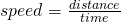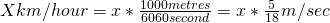If the ratio of the speed of A & B is a:b then the ratio of time taken by them to cover the same distance is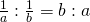“The time taken for covering a certain distance is increasingly proportional to the speed.”
Similarly time taken to finish a work is the inversed proportional to the number of working unit
When by A, cover a certain distance at x km/h & b cover the same distance at y km/h than the average speed of both during the whole journey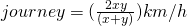Difference distance by two moving body in same direction with ‘u’ m/s and ‘v’ m/s
u>v then their relatives speed = (u-v) m/s
u>v in opposite direction they cross to each other =(With length of a & b meter)
u>v in same direction cross to each other =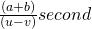if two bodies start at the same time from station A & b to words each other . they have taken time a & b second to reaching A to B & B to A .
then A’s speed: √b:√a